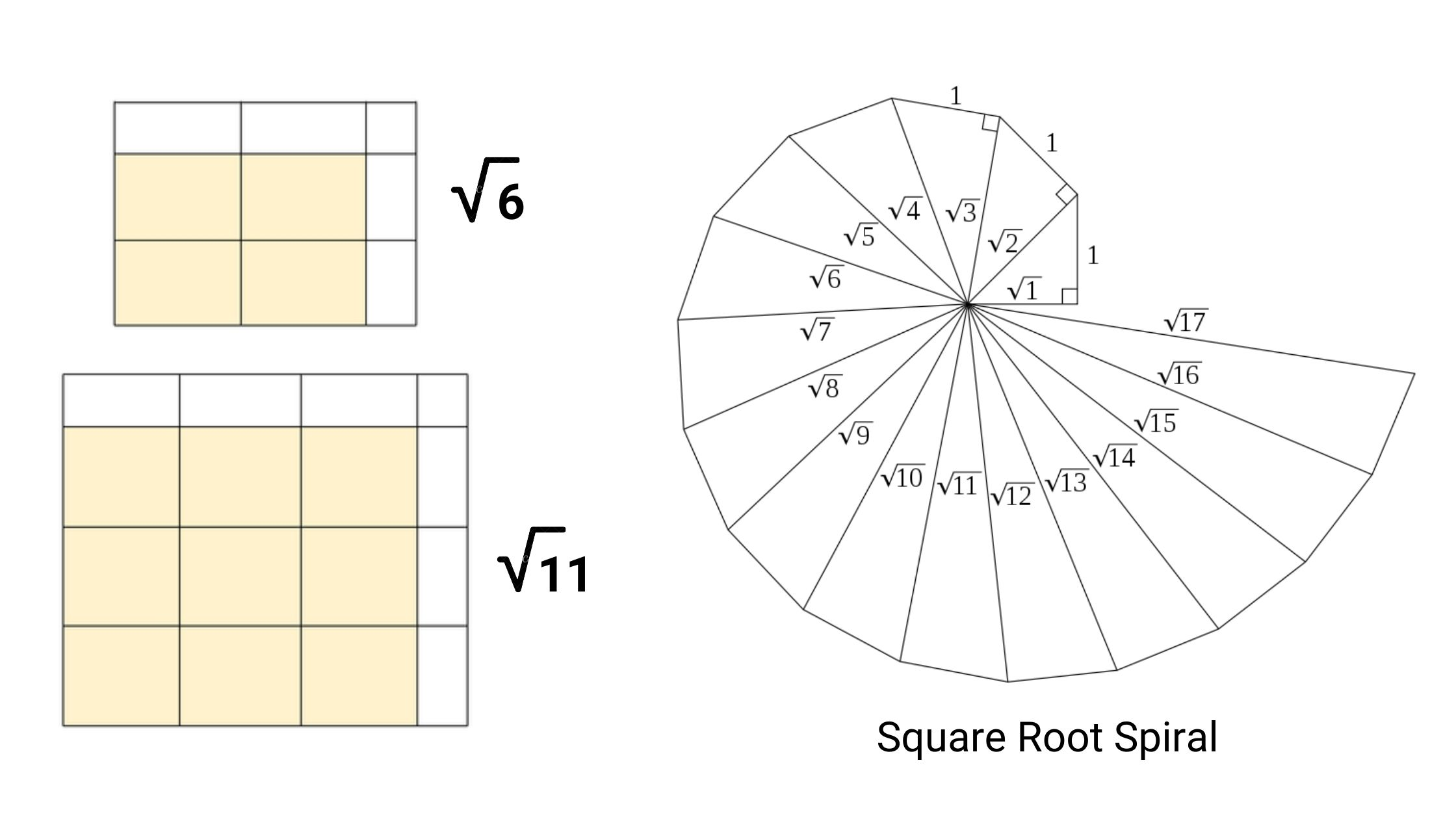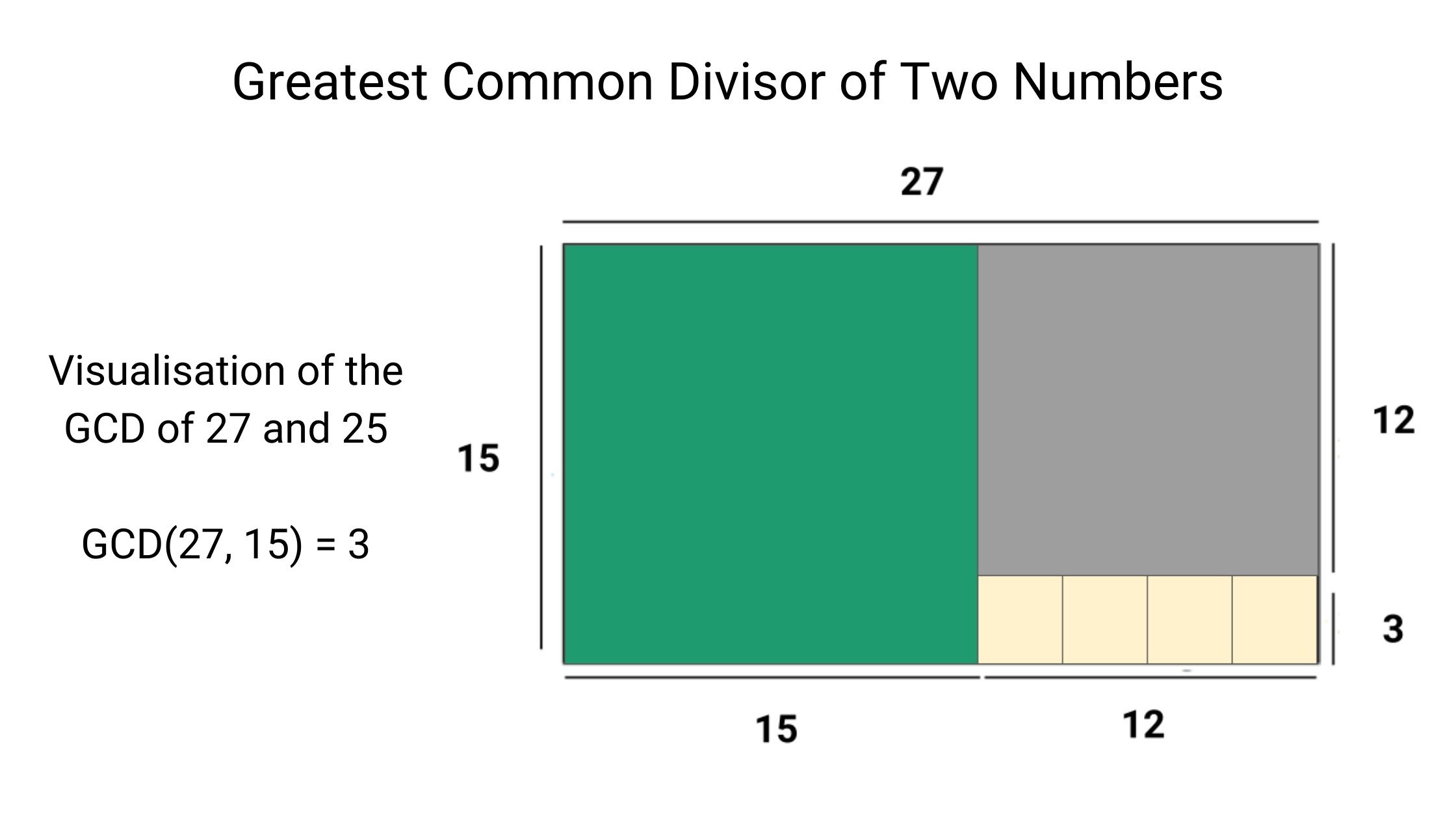# Enjoy Algorithmic Thinking

An initiative by enjoyalgorithms! We design content with in-depth explanations and help learners develop a dedicated interest in math, logic and algorithms.Find prime numbers in a range: Sieve of Eratosthenes

The sieve of Eratosthenes is an ancient and efficient algorithm for finding all primes numbers from 2 to n. This algorithm finds all the prime numbers in a segment using O(nlog⁡log⁡n) operations.Check if a number is prime or not: Primality Test

Given a positive integer n, write a program to check if the number is prime or not. A number n > 1 is said to be a prime number if 1 and n are its only factors. In other words, a prime number is a number that is divisible only by two numbers itself and one.Find Square Root of a Number

Given a natural number n, find the largest integer less than or equal to √n. This can be seen as a search problem where the search space S is the set {1, . . . , n}, and the number desired is the floor(√n), i.e., the largest integer that is less than or equal to √n.Find GCD of Two Numbers: Euclidean Algorithm

Given two non-negative integers, m and n, we have to find their greatest common divisor or HCF. It is the largest number, a divisor of both m and n. The Euclidean algorithm is one of the oldest and most widely known methods for computing the GCD of two integers.

© 2020 Code Algorithms Pvt. Ltd.